# Phong illumination model (cheat sheet)

$$f( \vec p ) = \underbrace{I_a K_a}_{\color{red} \text{ambient component}} + \underbrace{\sum_i^\text{nb_lights} (\vec n( \vec p ) . \vec l_i) K_d I_i}_{\color{green} \text{diffuse component}} + \underbrace{\sum_i^\text{nb_lights} f_{spec}(\vec l_i( \vec p ), \vec v(\vec p)) K_s I_i}_{\color{blue} \text{specular component}}$$

I summarize the Phong illumination model with the above equation and explain all the terms one by one.

French version: [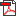pdf ] [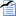odt ] Eclairage de phong résumé
$$\renewcommand{\vec}{ \mathbf{#1} }$$
The function $$f:\mathbb{R}^3 \rightarrow \mathbb R$$ takes a 3D point in input $$\vec p : (x,y,z)$$ and returns a real number which is the associated light intensity. $$\vec p$$ is a point on the object's surface where you'd like to compute the light intensity. Note that usually this function is evaluated 3 times for red, blue and green intensities. This sheet only describes the computation for one color channel.

Phong lighting is an extreme simplification of how lights really behaves, however, it is very efficient to compute.

### Ambiant component

In phong lighting we don't simulate light bouncing in the scene, but if we don't take this phenomenon into account, parts of the object not directly exposed to the source light would stay black. To fix this, the ambient component arbitrarily assigned a fixed intensity. This intensity only depends on the material of the object (assigned by the user)

• $$I_a$$ intensity of the scene (real number, user input)
• $$K_a$$ intensity of the material (real number, user input)

### Diffuse component (Lambertian diffusion)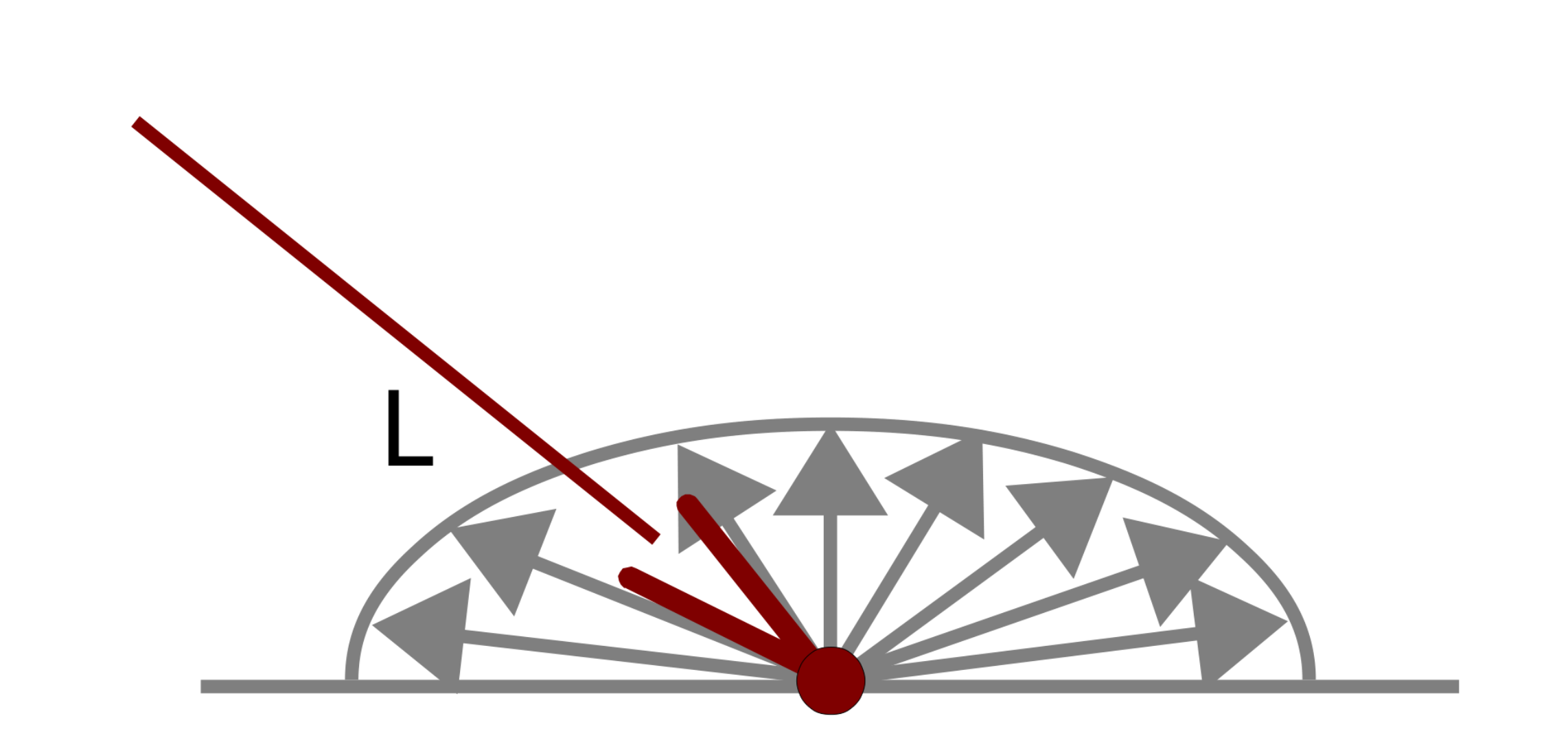Simulate the light that is scattered uniformly where it strikes the object's surface (e.g. plaster), it only depends on the light direction. The intensity is independent from the viewing point since light is diffused uniformly.

•  $$K_d$$ diffuse intensity of the material (real number, user defined)
• $$I_i$$ diffuse intensity of the ith light (real number, user defined)
• $$\vec n$$ normal vector at the position $$\vec p$$ on the object's surface (3D vector)
• $$\vec l_i$$ direction of the light striking at $$\vec p$$ (e.g. $$\vec l_i = \vec{lightPosition_i} - \vec p$$) (3D vector)

### Speculare component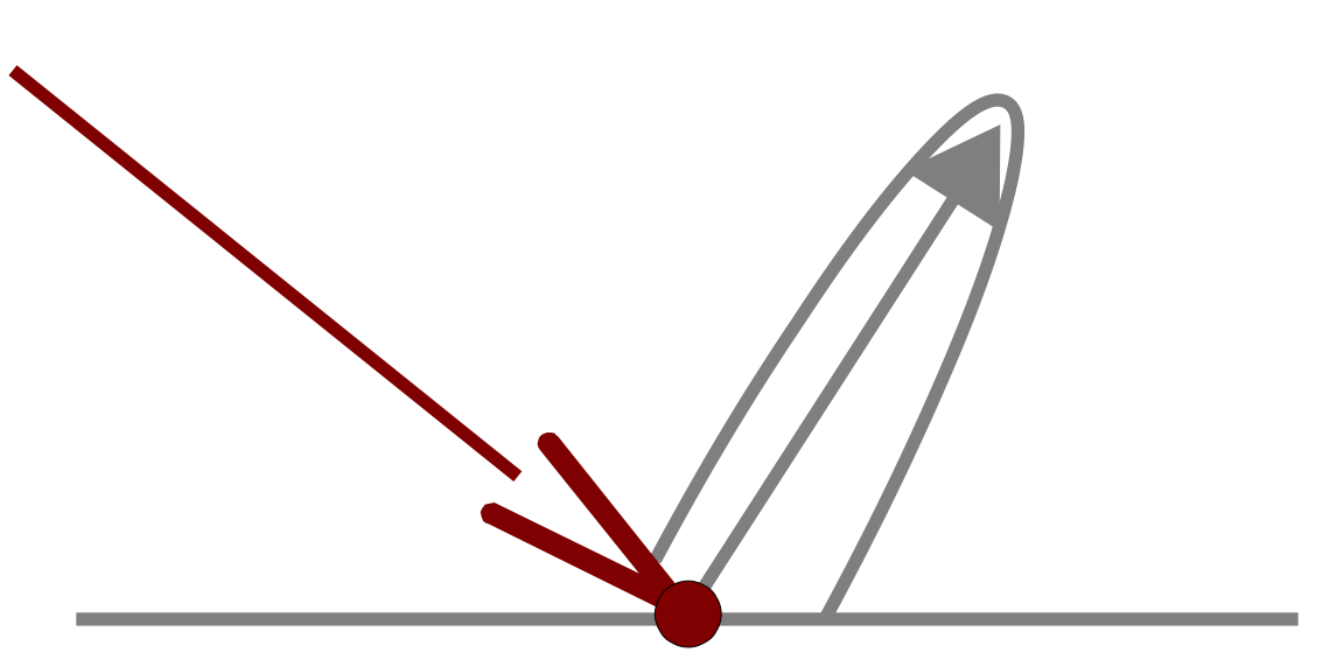Simulate light that is reflected off the object, therefor it depends on both the light source position and viewer perspective.

• $$f_{spec}(\vec l_i( \vec p ), \vec v(\vec p))$$ specular term described below (real number)
• $$\vec v$$ viewer direction (e.g. $$\vec l_i = \vec{cameraPosition} - \vec p$$) (3D vector)
• $$K_s$$ specular intensity of the material (real number) (user input)

#### Two ways to compute the specular term $$f_{spec}(\vec l_i( \vec p ), \vec v(\vec p))$$:

1) Phong's specular term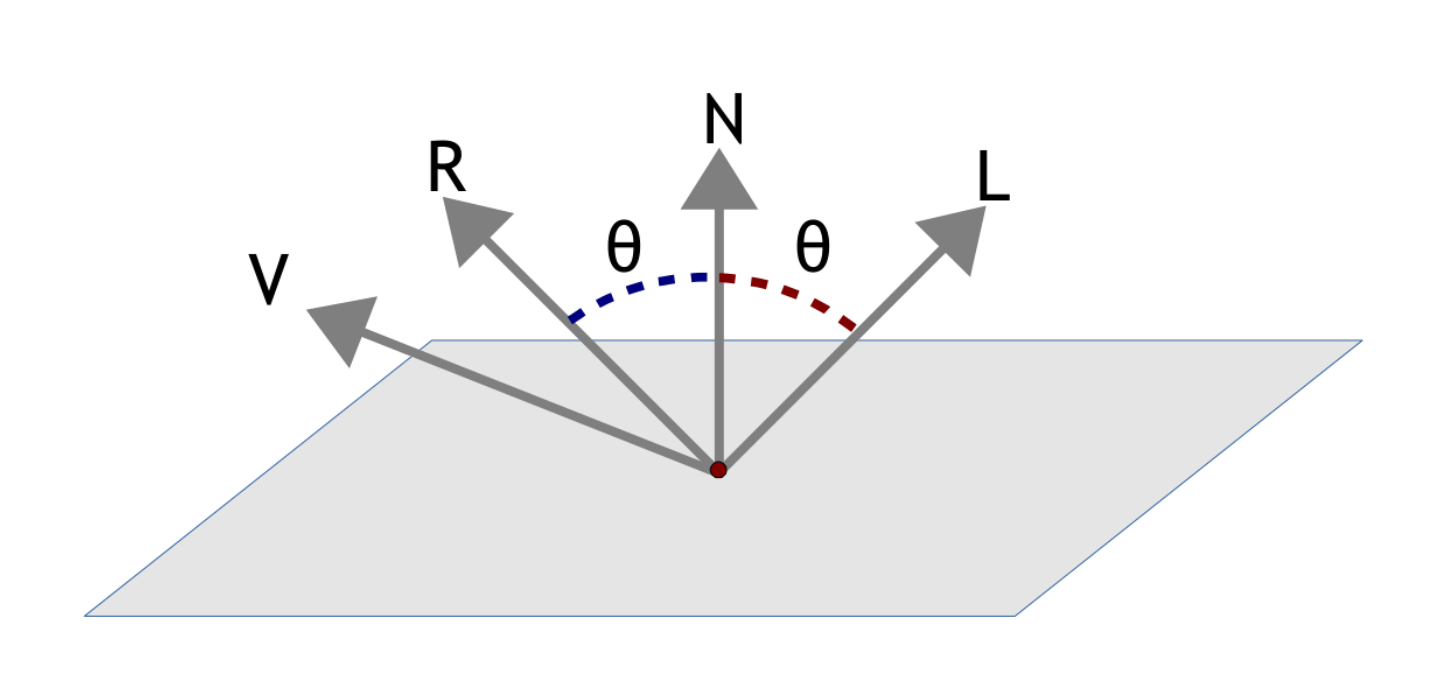$$\left \{ \begin{matrix} f_{spec} & = & (\vec r . \vec v)^c & \text{if } \vec r . \vec v > 0 \\ f_{spec} & = & 0 & \text{otherwise} \\ \end{matrix} \right.\$$

Where $$c$$ is coefficient representing the size of the specular reflection (user input) and $$\vec r$$ is the reflection of the light vector $$\vec l$$:

$$\vec r = (2(\vec n . \vec l) \vec n) - \vec l$$

2) Jim Blinn's specular term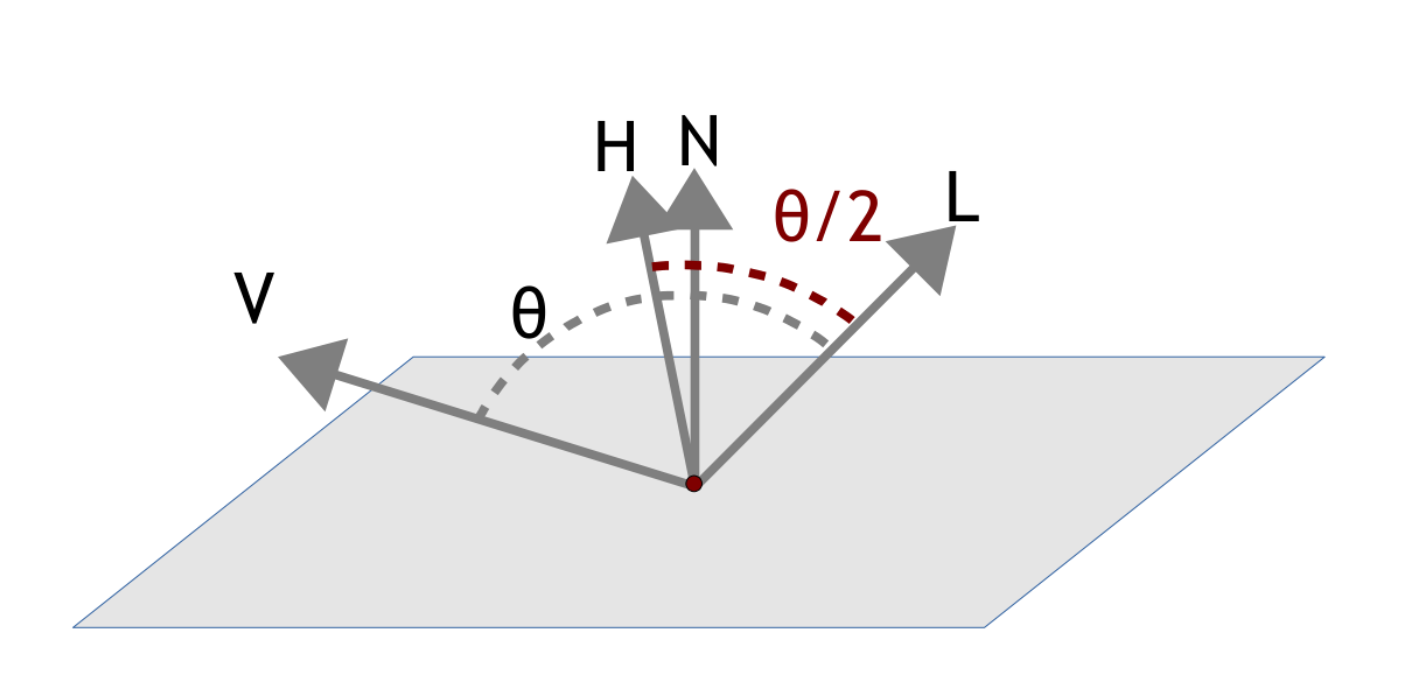$$f_{spec} = ( \vec n . \vec h)^c$$

Where $$\vec h$$ is the bisector between $$l$$ and $$\vec v$$:

$$\vec h = \frac{\vec l + \vec v}{ \| \vec l + \vec v \| }$$

References

See wikipedia's explanation.

One comment

Thanks for the post. There is a typo: the reflection should be r=(2(n.l)n)−l.
Rodolphe: Oh right thanks for noticing!

Warren - 14-11-’19 16:43
All html tags except <b> and <i> will be removed from your comment. You can make links by just typing the url or mail-address.
Anti-spam question: